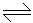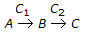# Chemical Engineering - Chemical Reaction Engineering

### Exercise :: Chemical Reaction Engineering - Section 5

1.

An isothermal aqueous phase reversible reaction, PR, is to be carried out in a mixed flow reactor. The reaction rate in k.mole/m3 .h is given by, r = 0.5CP - 0.125CR. A stream containing only P enters the reactor. The residence time required (in hours) for 40% conversion of P is

 A. 0.8 B. 1.33 C. 1.6 D. 2.67

Explanation:

No answer description available for this question. Let us discuss.

2.

Design of heterogeous catalytic reactor involves consideration of __________ steps.

 A. only chemical B. only physical C. both (a) & (b) D. neither (a) nor (b)

Explanation:

No answer description available for this question. Let us discuss.

3.

A consecutive reaction,, is characterised by

 A. maxima in the concentration of A. B. maxima in the concentration of B. C. maxima in the concentration of C. D. high exothermicity.

Explanation:

No answer description available for this question. Let us discuss.

4.

What is the unit of the rate constant in a chemical reaction in which 10% of the reactant decomposes in one hour, 20% in two hours, 30% in three hours and so on ?

 A. Litre/mole.second B. Moles/litre.second C. Litre/mole D. Litre/second

Explanation:

No answer description available for this question. Let us discuss.

5.

For a fluidised bed reactor, the most suitable/relevant model is a __________ model.

 A. tank in series B. bubbling bed C. plug flow D. none of these